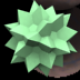# N Choose 2 is the Sum of the First N-1 Integers

Problem: Determine an arithmetic expression for $\binom{n}{2}$.

Solution: The following picture describes a bijection between the set of yellow dots and the set of pairs of purple dots:In particular, selecting any yellow dots and travelling downward along diagonals gives a unique pair of blue dots. Conversely, picking any pair of blue dots gives a unique yellow dot which is the meeting point (the “peak”) of the inward diagonals. If we say the bottom row has $n$ elements, then the number of yellow dots is clearly $1 + 2 + \dots + (n-1)$, and the number of pairs in the last row is just $\binom{n}{2}$.

This proof is typical of the most elegant combinatorial proofs: come up with a bijection between one set of objects whose size is unknown and another set of objects whose size is easy to determine. Such a method of proof is ubiquitous in elementary combinatorics, and it extends even further to determine things about infinite sets. In higher mathematics, bijections are quite frequently used (along with other structure-preserving conditions) to determine when two things should be deemed equivalent. This concept is called isomorphism, and much of mathematics is devoted to classifying all objects in a category up to an appropriate notion of isomorphism. One particularly long instance of this is the classification of finite simple groups, but one which is easier to understand, and studied extensively by mathematicians since time immemorial, is the classification of Euclidean isometries (see here for the case of $\mathbb{R}^2$).

## One thought on “N Choose 2 is the Sum of the First N-1 Integers”

1.Gaston Bengolea

I love your blog, and this entry is very nice and self-explanatory!